﻿ Numerical Modeling of the Behavior of a Pile Foundation under Axial Load with AnsysPublications are Open
Access in this journal
Article Versions
Export Article
• Normal Style
• MLA Style
• APA Style
• Chicago Style
Research Article
Open Access Peer-reviewed

### Numerical Modeling of the Behavior of a Pile Foundation under Axial Load with Ansys

Oustasse Abdoulaye SALL , Déthié SARR, Makhaly BA, Atsou Edem TCHAKOU
American Journal of Materials Science and Engineering. 2019, 7(1), 12-20. DOI: 10.12691/ajmse-7-1-3
Received October 15, 2019; Revised November 24, 2019; Accepted December 12, 2019

### Abstract

This paper studies the behavior of an isolated pile under static axial load and aims to analyze this type of foundation taking into account the soil-structure interaction. The implemented approach is a finite element analysis of a numerical behavior model of the soil-pile system, with emphasis on the behavioral parametric study of the system. A three-dimensional numerical model taking into account the geometric, mechanical and geotechnical parameters of the soil was used for modeling on Ansys using finite element method (FEM). This allowed us to study the influence of soil behavior (elastic or elastoplastic perfect) on the response of the pile under axial load. The study also shows that the increase of the soil reaction module gives rise to a dome effect or a much more pronounced thrust in the elastoplastic domain at a certain depth. The study also shows an increase of the reaction module of the soil mass with the depth and consequently a decrease of the deformations.

### 1. Introduction

In the study of civil engineering works, the mastery of the behavior of foundations plays a key role. The characterization of the foundations can not be done reliably without an adequate consideration of the soil-structure interaction.

The phenomenon of modification of the soil response due to the presence of the structure is called soil-structure interaction. At present, in the "engineering practice", this phenomenon of interaction is not rigorously taken into account during the design of foundation. However, in the current state of the art, should we allow ourselves to ignore the phenomenon of soil-structure interaction? Today, are the geotechnical and structural calculations to be carried out separately?

As studies 1, 2, 3 have shown the influence of soil properties on the behavior of mat foundations (and reciprocally). A recent study 4 has shown in the case of an analytical calculation the influence of the mechanical properties of the soil mass on the behavior of deep foundations. It is in this context that to deepen the study, we are interested in the numerical modeling of the soil-structure interaction in the case of deep foundations. In the study of soil-structure interaction problems, the horizontal response of a soil on a pile is often modeled by interaction laws that more or less accurately represent reality. The soil is not perfectly rigid, under the effect of a load; the foundation and the superstructure react simultaneously and interact with each other. The reaction of the superstructure causes forces on the foundation that are transmitted to the ground, thus modifying its response.

As part of this study, the modeling of the soil-pile interaction will be presented at first. For the rest, it will be presented the mechanical behavior model of isolated piles under axial load, their numerical modeling under Ansys and will end with a discussion of the results.

### 2. Methodology

In this study, it will first be necessary to characterize the soil mass in order to elaborate the model of behavior of the soil-pile interface and determine the input parameters. For the purposes of the study, the contact zones are represented by thin-layer interface elements characterized by horizontal and vertical translation (Kh and Kv) and torsion (K) rigidities. These reaction modules depend on the mechanical properties of the foundation soil, the geometric and mechanical characteristics of the foundation.

The behavior of the soil-pile system is governed by a Mohr-Coulomb behavior law. A numerical simulation of the soil-pile system will be carried out on Ansys to study the influence of the parameters of the interface on the behavior of foundation for various laws of behavior of the soil mass.

2.1. Modeling of the Soil-Pile Interaction
2.1.1. Spring-type Interface Element

The soil behavior is modeled by a set of springs infinitely near each other (Figure 1) defining the coefficients of proportionality between the reactions of the soil and the components of the displacement of the foundation.

These experimental or empirical coefficients are, however, applicable only in a very limited field of dimension and load on foundation. A precise characterization of these springs was made by considering the soil as a homogeneous elastic semi-space (semi-infinite elastic medium). The problem has been studied in the case of circular foundation according to the methods of Deleuze 5 and Newmark-Resenblueth 6. For other types of superficial foundations, rigidities may be determined by the Cavaer-Sieffert method 7, 8.

The definition of the soil-structure interface is a very important parameter in the study of the behavior of foundation structures. In the study, the soil is modeled by a set of springs whose shear modulus Gs (Table 1) and parameters βx, βz and β depend on the section of the foundation and make it possible to characterize the soil-pile interface. For the determination of shear modulus and stiffness, the following formula 6 will be used: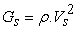(1)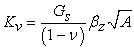(2)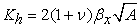(3)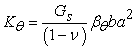(4)

with:

Vs : shear rate given by Table 1,

Kv : vertical translation rigidity;

Kh : horizontal translation rigidity;

Kθ : rigidity of rotation;

βx, βθ, βz parameters depending on the ratio a/b, a and b respectively the length and the width of the foundation;

A: the area of the section of the foundation.

With if

Ÿ a/b =1 so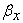= 1,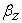=2.16,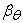=0.5

Ÿ a/b =2 so=0.94,=2.2,= 0.6

Ÿ a/b =4 so= 1,=2.4,= 0.79.

2.1.2. Numerical Modeling of Contact by the MEF

Many authors 10, 11, 12, 13, 14, 15, 16, 17, 18, 19, 20, 21, 22, 23 have proposed the basic principles of the finite element method and its application for solving problems such as the study of pile behavior. Several methods for modeling discontinuous behavior at the soil-structure interface have been proposed:

Ÿ The use of "zero-thickness elements" with two nodes where one considers as active only the connection between the opposite nodes (Figure 2.b).

Ÿ The use of be-dimensional or three-dimensional "thin-layer elements" by assigning to these behavior laws derived from the theory of the elastoplasticity (Figure 2.d).

Ÿ Hybrid methods where soil and structure are modeled separately and then linked by different compatibility equations for forces and displacements. With the elements "without thickness", the continuity of the displacements with the interface is ensured by the application of a method of penalization or adaptation of the rigidity and the condition of relative sliding is controlled by the Mohr-Coulomb criterion.

• Figure 1. Spring-type interface element
• Figure 2. Digital discretization of the soil-structure problem 
2.2. Solving Contact Problems with the FEM

In the resolution of problems with boundary conditions integrating the interfaces, the use of finite elements for the modeling of the contact with usual elements poses problems because the relative movement of the solids in contact is not compatible with the condition of continuity of displacement required at nodes of adjacent elements.

Soil-pile interaction is a typical example of an interface problem. It is emphasized that a particular difficulty exists in the case of piles: it is about the definition of the initial state in the ground and the interface, problem far from being solved, considering the difficulties of correct simulation of the setting up piles (simulation of threshing, drilling, etc.). Conventional pile calculation methods are based on empirical correlations leading to the determination of the limiting bearing capacity. In most cases, relatively less attention is given to determining the deformation characteristics that correspond to the service phase of a pile.

However, recent design and calculation recommendations for deep foundations at the European zone (Eurocode 7) suggest the calculation of settlements and horizontal displacements as excessive values of these can affect the functioning of the structure.

2.2.1. Mesh of an Isolated Pile

The analysis by finite elements requires the realization of a mesh and the taking into account of the conditions to the limits of the studied field: displacements, stresses, interstitial pressures or imposed temperatures. These conditions may be at a "finite" distance; bond or rigid substratum, obstacle preventing a displacement or "infinite"; zero displacement to infinity imposed state of constraints 25.

Most often, meshes are built only with finite elements; this is possible because the effects of static loading decrease rapidly with the distance from the application point (or zone). Parametric studies then make it possible to determine the minimum extent of the medium to be modeled so that the effect of the loadings is not disturbed by these boundary conditions.

• Figure 3. Boundaries of the domain 

2.2.2. Boundary Conditions

The mesh of an isolated pile, placed in a semi-infinite homogeneous soil mass (Figure 3), must be realized taking into account the following recommendations 25:

Ÿ the vertical limits of the mesh (condition u = 0) must be set at least twice the length L of the pile and the lower horizontal limit (condition v = 0 or u = v = 0) to at least twice the length L at the below of the tip (3L from the surface), so that the boundary conditions in displacement do not have any influence on the behavior of the pile,

Ÿ The interfaces between the pile and the ground (barrel and tip) are represented by finite interface elements compatible with the massive elements used to describe the soil;

Ÿ The mesh of an isolated pile must be relatively tight in the vicinity of the interfaces, that is to say at the point and along the shaft, where strong gradients may appear;

Ÿ The same refinement should exist under the point in the vertical direction towards the substratum or the lower limit condition;

Ÿ Aspect ratio (ratio of the smallest dimension of an element to the largest) of the elements near the pile should be limited to 1/5;

Ÿ The width B (in the horizontal direction) of the first element directly adjacent to the pile must be at least such that B = 0, ld, where d is the diameter of the pile.

Ÿ The pile used will be free in the lead and anchored to the point

### 3. Simulation under Ansys of the Soil-Pile System

3.1. Calculation Approach on Ansys

Ansys is a highly sophisticated finite element program designed primarily to model the behavior of solids and externally applied load structures. The calculation procedure is given in Figure 4 below.

In this study, finite element analysis on Ansys can be summarized in three main steps:

Pretreatment

Ÿ Enter the type of element

Ÿ Enter the properties of the material (Young's modulus, Poisson's ratio, density ...)

Ÿ Enter the geometrical data (thickness of the plates, surfaces, volume, inertia ...)

Ÿ Generate the mesh

Ÿ Enter the load

Ÿ Enter the boundary conditions (supports, cancel deformations ...)

Resolution

Ÿ Setup

Ÿ Solution

Post-treatment

Ÿ Listing

Ÿ Visualization of the results

Ÿ Importing results

3.2. Presentation of the Digital Model

At first, the soil mass and the pile (concrete) are considered to have an elastic linear behavior. For the purposes of the simulation, we consider a circular pile of 50 cm diameter and ten meters long subjected to axial loads ranging from 100 to 500 kN. For parametric analysis, we will make the geometric and mechanical characteristics of the soil-pile system. Figure 5 shows the mesh of the problem.

• Figure 4. Design cycle
• Figure 5. Mesh of the geometric mode
• Figure 6. Displacements obtained for lateral loading

### 4. Presentation of the Results

The next section, will present the results for pile (recessed tip and free head) subject to a vertical load of 500 kN with mechanical characteristics given in Table 2. The results presented in Figure 6 show that the compression under axial load causes a deflection in a direction perpendicular to the axis of compression. Which means that in fact, even under axial load, the pile is loaded with compound bending and not with simple compression.

From Figure 6 it can be seen that the greatest displacements along the pile occur at the head. It shows that the displacements remain almost constant for depths Z ≥ 6m, but they progressively increase for depths Z <6m, whatever the loading value adopted. The analysis shows that loading effects (in terms of displacements) are felt on the upper third of the pile. These results are in phase with those obtained by 4 based on an analytical calculation.

4.1. Case of a Linear Elastic Soil
4.1.1. Influence of the Young's modulus (Eb) of the pile

In this section, it is proposed to study the behavior of the pile for various values of its Young's modulus with the characteristics of the soil mass recorded in Table 3. The results are given by Figure 7 to Figure 9.

Figure 7 to Figure 9 show, in addition to the deflection (Figure 6), a compression of the pile for an axial load of 500kN applied at the head. The results show that the different sections do not have the same sensitivity to loading because the closer the section is to the point of application of load, the greater its displacement. The study also shows that any pile subjected to axial loading tendency to flex and deform along an axis perpendicular to the axis of compression. The results show that the higher the Young's modulus of the pile, the lower the axial displacements. The maximum value of these displacements varies from 1.15mm to 0.61mm when the Eb module increases from 20 to 40 GPa. The results also show that for an Eb module equal to 20 GPa, the maximum deflection is of the order of 0.75mm and that for a value of 40 GPa of this module, the deflection is equal to 0.35mm.

• Figure 7. Vertical displacement of the pile for Eb = 20.000MPa
• Figure 8. Vertical displacement of the pile for Eb = 30.000MPa
• Figure 9. Vertical displacement of the pile for Eb = 40.000MPa

3.1.2. Influence of the Soil Reaction Module

In this part, the load is always set at 500kN and the pile module at 35GPa. We will vary the modulus of elasticity of the soil between 15 and 23 MPa to study the influence of this parameter on the pile response. The characteristics of the materials are given in Table 4. The results obtained are given by FIGS. 10 to 12.

• Figure 10. Vertical displacement of the pile for Es=15 MPa
• Figure 11. Vertical displacement of the pile for Es=20 MPa
• Figure 12. Vertical displacement of the pile for Es=23 MPa

Note that despite the increase in the soil reaction module from 15 MPa to 23 MPa, the difference between axial displacements is almost zero (Figure 11 and Figure 12). The results show that the soil reaction module has a very weak influence on the axial displacements because it is noted that these displacements are almost identical regardless of the value of the modulus of elasticity of the soil (Figure 11 and Figure 12).

4.1.3. Influence of axial load

In this section, we study the evolution of pile deformations for various Q load values ranging from 400kN to 600kN. The characteristics of the materials are given in Table 5. The results obtained are given in Figure 13 to Figure 15.

• Figure 13. Vertical displacement of the pile for Q=400kN
• Figure 14. Vertical displacement of the pile for Q=500kN
• Figure 15. Vertical displacement of the pile for Q = 600kN

The analysis of the results of the compression and the deflection of the pile for various values of the load Q shows an increase of these values with those to the load. As the load increases, displacement increases.

4.2. Case of Elastoplastic Soil

Even if most computational software admits a linear behavior of the ground foundation, in the reality of the facts, it is rarer to meet soils having an elastic behavior. We will therefore, in what follows, resume the previous study by giving the ground an elastoplastic behavior (Figure 16 and Figure 18).

4.2.1. Influence of Pile Young Modulus (Eb)

Table 6 below gives the characteristics of the materials for the parametric study for various values of Young's modulus of concrete.

• Figure 16. Vertical displacement of the pile for Eb=20.000MPa
• Figure 17. Vertical displacement of the pile for Eb=30.000MPa
• Figure 18. Vertical displacement of the pile for Eb=40.000MPa

As in the case of resilient soils, we note that the higher the Eb module of the pile, the more the displacements decrease. It can be seen that in the elastoplastic domain, the displacements are greater than those observed in the elastic domain. In the elastic domain, the deflection of the pile is limited to 0.75 mm whereas in the elastoplastic field the limit value is equal to 0.60 mm at the head. This means that in the elastoplastic field, the ground mobilizes more horizontal resistance than in the elastic domain. The results also show that in the elastic domain, the "neutral point", that is to say, the point insensitive to loading (displacement following y is zero) is in the upper half of the pile while in the elastoplastic domain, this neutral point in the second lower half. In the next lines, we will vary the value of the Es module of the soil to evaluate its influence.

4.2.2 Influence of the Soil Reaction Module

Table 7 below gives the characteristics of the materials for the parametric study for various values of the Young's modulus of the foundation soil (Figure 19 and Figure 21).

• Figure 19. Vertical displacement of the pile for Es=15MPa
• Figure 20. Vertical displacement of the pile for Es=20MPa
• Figure 21. Vertical displacement of the pile for Es=23MPa

The results show that the variations of the modulus Es of the soil cause a small variation of displacements in the three cases.

This variation is felt to a depth of six meters. And beyond this depth, the displacements are almost identical. This weak influence on the deformations is due to the behavior of the soil mobilized around the pile. What is important to note is the "dome effect" obtained at 4 meters for the three different modules. This can be explained by the increase of the soil reaction module as a function of depth. Beyond that of 4 meters, the pile is weakly solicited. So we can think that the soil reaction module is little mobilized peak.

### 5. Conclusion

This article deals with the study of the behavior of an isolated pile under static axial load and aims to analyze this type of foundations under axial load by taking soil-structure interaction. The approach implemented is the finite element study of a numerical model of the soil-pile system, with emphasis on the parametric behavioral study of the system. To reach our goal, we used a finite element code, Ansys, to make a three-dimensional modeling of the soil mass in which a pile (considered as beam) free at the top anchored in point is implanted. A series of analyzes was conducted to study the influence of parameters such as the soil reaction module (Es) and the Young's modulus (Eb) of the pile or the load Q on the behavior of a pile subjected to a static axial load in the elastic and elastoplastic domain. The analysis of the results obtained shows a much greater influence of the reaction module on the behavior of the pile in the elastoplastic domain (Mohr model) for the sand.

The analysis of the deflection of the pile leads us to conclude that there is a variation of the soil reaction module as a function of the depth. This influence is felt more in the upper half-thickness of the soil mass. Beyond this depth, the importance of this effect diminishes. At the end of the study, we were able to remember that:

Ÿ An increase in the soil reaction modulus causes a decrease of the displacements of the pile, this shows that the soil mass participates in the resistance of the pile.

Ÿ When a free-standing pile anchored at the top is axially stressed at the head, the greatest displacement value is located at the level of the head, whatever the nature of the soil, the law of behavior with which this soil is modeled (elastic or elastoplastic) as well as the applied load.

Ÿ The effect of the reaction module is felt more in the upper half-thickness, this shows an increase of the reaction module with the depth. This can be explained by the fact that the deeper we go, the denser the soil becomes, the more the effective stress increases. An increase in the effective stress results in an increase in the horizontal stress, that of the horizontal reaction module.

The deflection along the pile which passes from a positive value to a negative value highlights a phenomenon of abutment and counter stop along the pile.

### References

  Sall, O.A., Ba, M., Ndiaye, M., Sangare, D., Fall, M. and Thiam, A. (2015), ‘‘Influence of Mechanical Properties of Concrete and Soil on Solicitations of Mat Foundation. Open Journal of Civil Engineering, 5, 249-260. In article View Article  Sall O.A Fall M., Berthaud Y., Ba M., ‘‘Influence of the Elastic Modulus of the Soil and Concrete Foundation on the Displacements of a Mat Foundation’’, Open Journal of Civil Engineering, 2013, 3, 228-233. In article View Article  Sall O. A., Fall M, Berthaud Y., Ba M, Ndiaye M., “Influence of the Soil-Structure Interaction in the Behavior of Mat Foundation”, Open Journal of Civil Engineering, 2014, 4, 71-83. In article View Article  Sall O.A., Ba M., Sarr D, Ngom D., Seye A., ‘‘Prise en compte de l’interaction sol-structure dans l’étude du comportement des pieux sous charge axiale’’ Afrique SCIENCE 13(6) (2017) 435-445. In article  Deleuze G., “Réponse à un mouvement sismique d’un édifice pose sur un sol élastique “Annale de l’ITBTP n° 234, Paris. 1967. In article  Newmark NM, Rosenblueth E Fundamentals of earthquake engineering. Prentice-Hall: Englewood Cliffs, Nj, 1971. In article  Sieffert J-G, Cevaer F., “Manuel des function d’impédance- Fondations superficielles, Ouest’’, Paris. 1967. In article  Sieffert J.–G., Cevaer F. (1992), “Manuel des fonctions d’impédance, fondations superﬁcielles”, Ouest éditions, Presses Académiques, France, 173 pages. In article  Jendoubi A. (2015). Effet de l’interaction dynamique linéaire et non-linéaire sol-structure: application aux pylônes de transport d’énergie, Thèse de Doctorat, spécialité Génie Civil, 126 pages. In article  Poulos H G (1971). Behavior of laterally loaded piles: I-Single piles. Journal of the soil Mechanics and Foundations Division, ASCE, vol 97, SM5, Proc. Paper 8092, May, pp 711731. In article  Schreyer H.L., Shih PY. (1973). Lower bounds to column buckling loads. J. of Eng Mech. Div, Proceedings of ASCE, vol. 18, pp. 1011-1022. In article  AMS AM 00 J D V (1975). Buckling capacity of piles in soft clay. J. Geotechn Engng Div, Proc ASCE. vol 101. n° GTI 1. Tcchn N., pp 1187-1191. In article  Davisson H.L., Donovan T.D. (1977). “Design approach for laterally loaded drilled piers”, GAI report to Pennsylvania Power and Light Compagny, December. In article  Baguelin F., Frank R., Saïd Y.H. (1977), “Etude théorique du mécanisme de réaction latérale des pieux”. Bulletin de Liaison des Laboratoires des Ponts et Chaussées, n° 92, pp. 35-55. In article  Coin A., Albiges M. (1978). “RDM appliquée, Flambement et Instabilité élastique”, Eyrolles, pp.575. In article  Poulos HG. Davis EH (1980) ‘‘Pile foundation analysis and design Senes in geotechnical engineering’’, J Wileyand sons editors/ In article  Souche P. (1984), ‘‘Etude du flambement de pieux partiellement immergés dans un milieu offrant latéralement une réaction élastique pure’’ Annales IT BT.P., n° 423, pp. 38-60. In article  Sall O. A., Ba M, Sarr Déthié, Ngom D. et S. M. A. (2017) ‘‘ Prise en compte de l’interaction sol-structure dans l’étude du comportement des pieux sous charge axiale’’ Afrique SCIENCE 13(6) (2017) 435-445, ISSN 1813-548X, http://www.afriquescience.info. In article  Perie F. (1986), “Etude numérique du flambage des pieux du quai de Lorien", Rapport technique de la société ESI (Engineering System International). In article  Vezole P. (1989), “Stabilité de forme des micropieux”, Annales de l’ITBTP, N° 478. In article  Pantelides C.P. (1990), “Stability of columns on biparametric foundations. Computers & Structures, vol. 42, n° 1, pp. 21-29. In article View Article  Emile Youssef., (1994), “Etude theorique et experimentaledu flambement des pieux”, Thése de doctorat. Ecole Nationale des Ponts et Chaussées, 271 p. In article  De Gennar V. & Frank R., (2005), ‘‘Modélisation de l’interaction sol-pieu par la méthode des éléments finis’’. Ed. CERMES. In article  David M. Potts, Lidija Zdravković, ‘‘Finite Element Analysis in Geotechnical Engineering’’, Theory Volume 2 of Finite Element Analysis in Geo · Finite Element Analysis in Geotechnical Engineering, Edition, illustrated. Publisher, Thomas Telford, 1999. ISBN, 0727727532, 9780727727534, 440 pages. In article  Mestat P. (1997). “Maillages d'éléments finis pour les ouvrages de géotechnique Conseils et recommendation” bulletin des laboratoires des ponts et chaussées - 212 - Novembre-Décembre 1997, 26 pages. In article

Published with license by Science and Education Publishing, Copyright © 2019 Oustasse Abdoulaye SALL, Déthié SARR, Makhaly BA and Atsou Edem TCHAKOUThis work is licensed under a Creative Commons Attribution 4.0 International License. To view a copy of this license, visit http://creativecommons.org/licenses/by/4.0/